# 如何安装并使用 OpenCL 计算

25 三月 2014, 07:12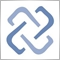0
12 191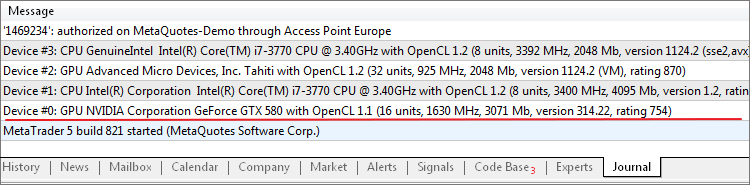### 什么是 OpenCL?

OpenCL 是由 Khronos Group 集团于 2008 年开发的并行编程开放标准。这一标准允许您利用异构系统中的不同架构，开发可以在 GPUCPU 上并行运行的应用程序。

### OpenCL 支持

MQL5 中对于 OpenCL 的支持始于 2010 年 6 月发布的 1.1 版本。所以，为了使用并行计算，您需要拥有一种适合此标准的较新的软件和硬件。

Intel CPU：

Core i3, i5, i7 - 用于 PC；
Xeon - 用于服务器；
Xeon Phi - 用于协处理器 （了解更多)。
Windows 7, 8;
openSUSE;
Red Hat.
AMD 显卡：

6400 系列及以上版本的 AMD Radeon HD 显卡；
5400 系列及以上版本的 ATI Radeon HD 显卡；
ATI FirePro 显卡 A300、S、W、V 系列；
5400 系列及以上版本的 ATI Mobility Radeon HD；
ATI FirePro M7820 M5800 (read more)。

Opteron、Athlon 64、Athlon 64 FX、Athlon 64 X2、Sempron、Turion 64、Turion 64 X2、Phenom、Phenom II （了解更多）。

APU （混合型 CPU/GPU 处理器）：

A、C、E、E2、G、R 系列 CPU。
Windows Vista SP2, 7, 8；
openSUSE 11.x；
Ubuntu 11.04；
Red Hat 6.x。
NVidia GPU （带有 CUDA 架构）：

Windows XP、Vista、7、8
Linux 以及 Mac 操作系统

### 1. Intel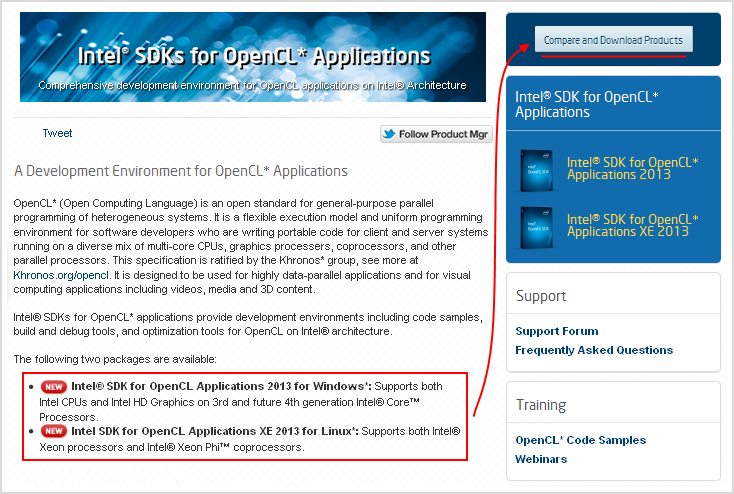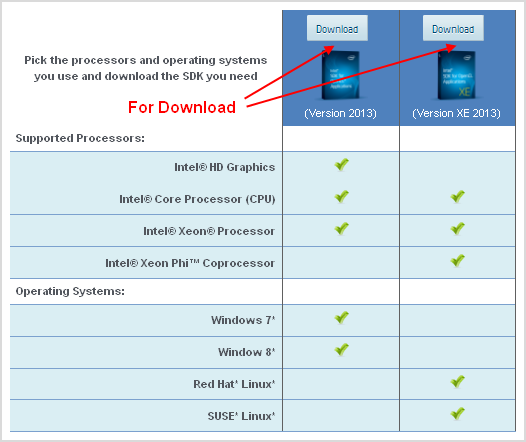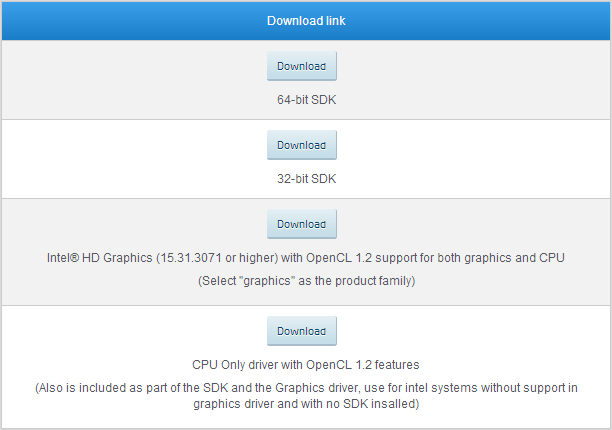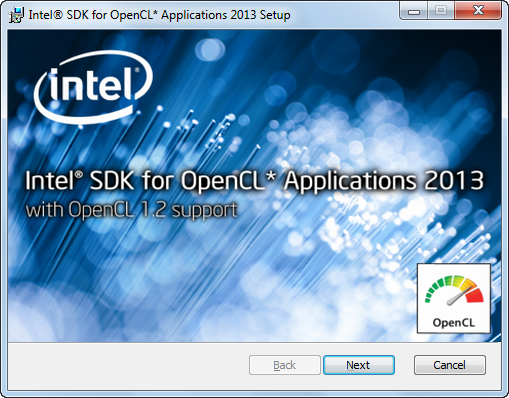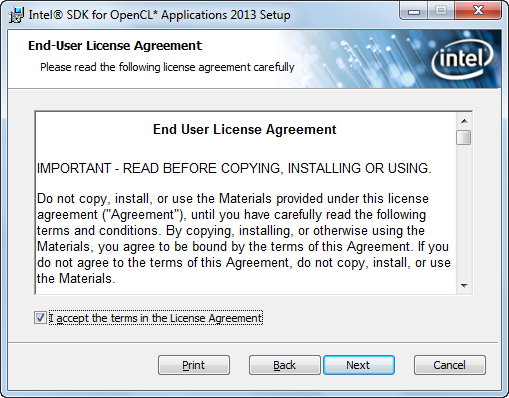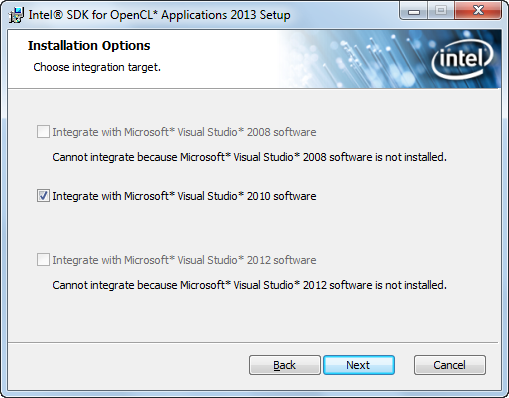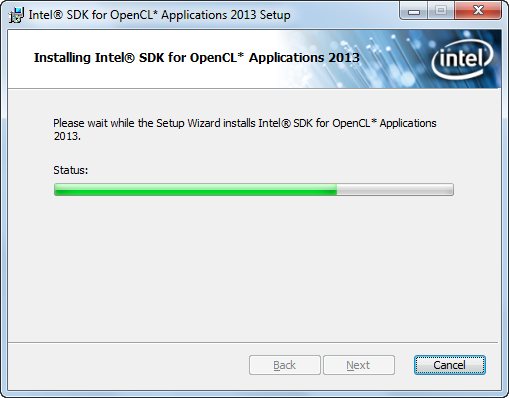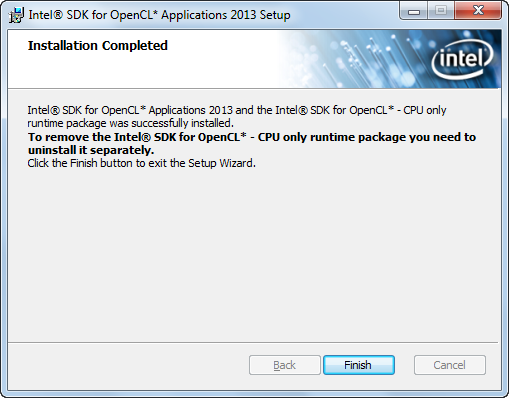### 2.1. AMD 显卡与 APU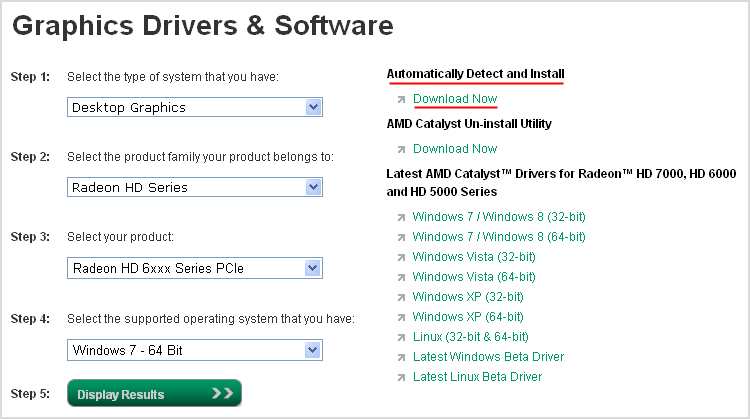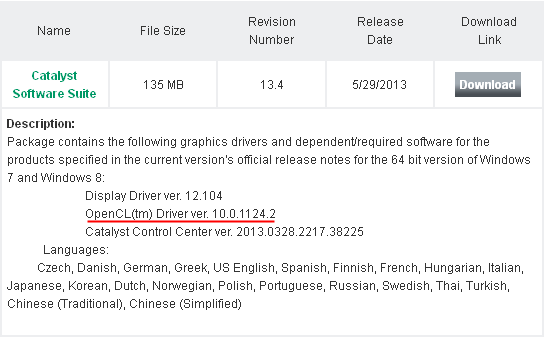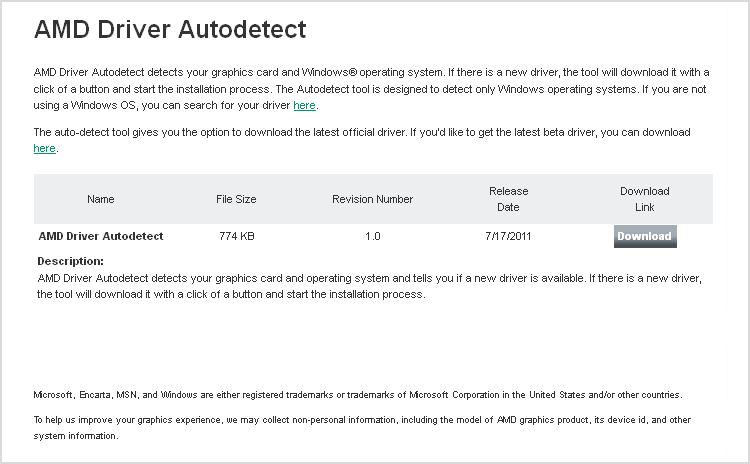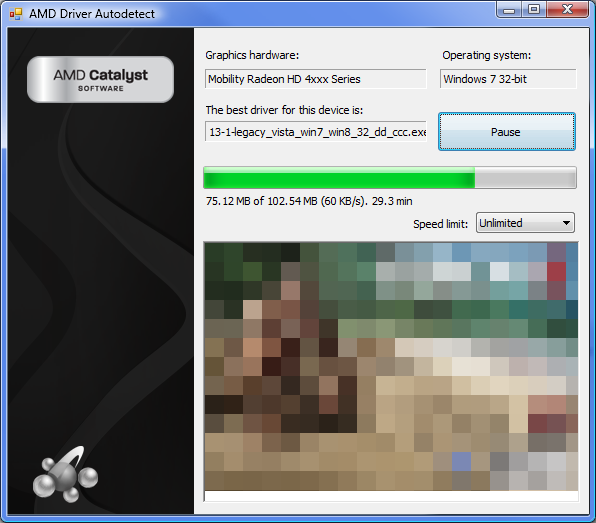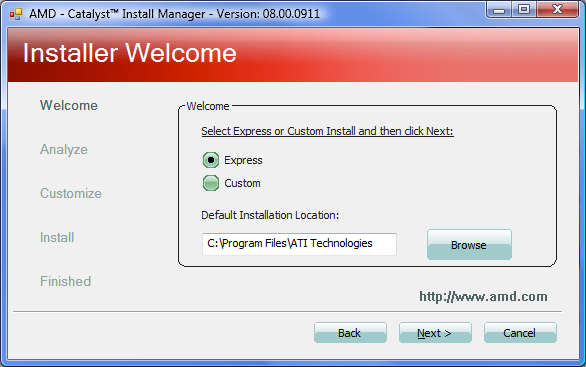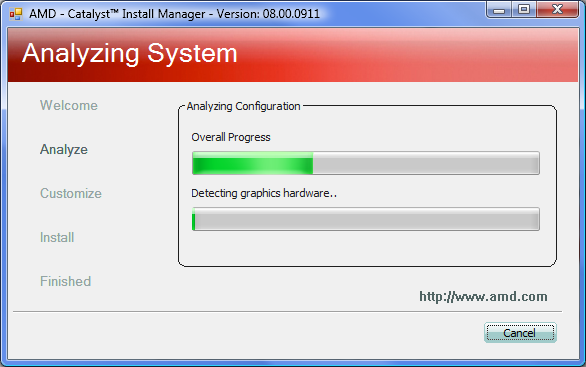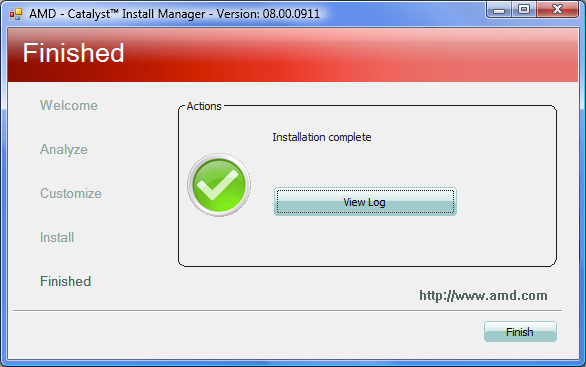### 2.2. AMD CPU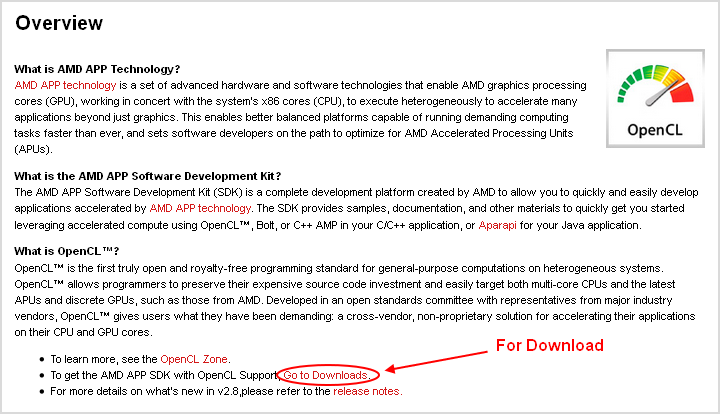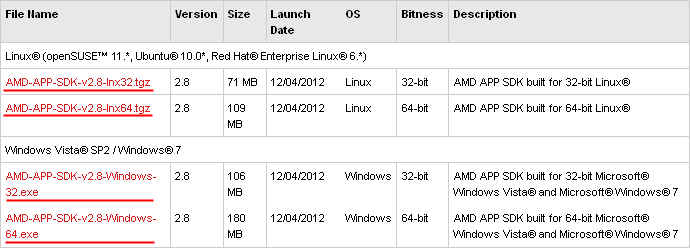### 3. NVidia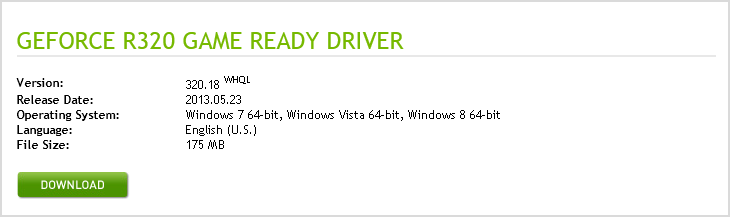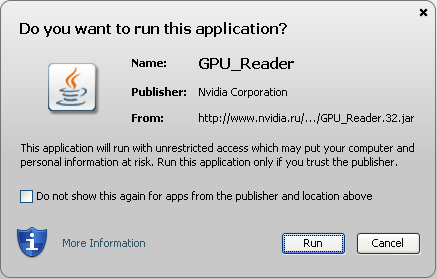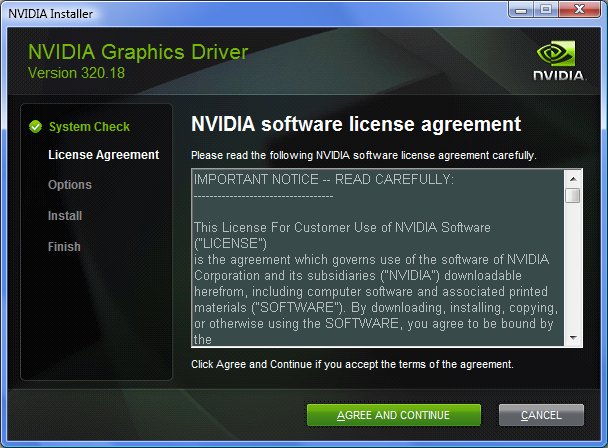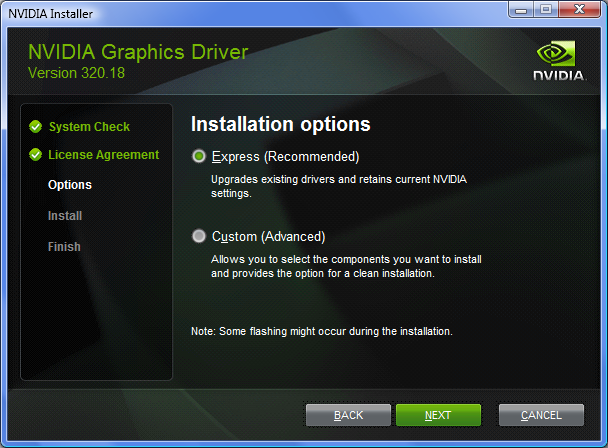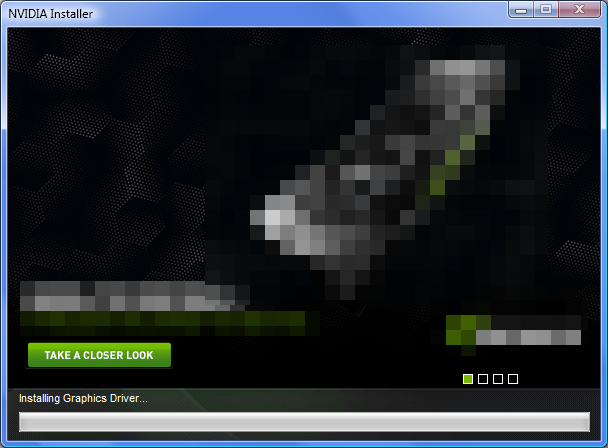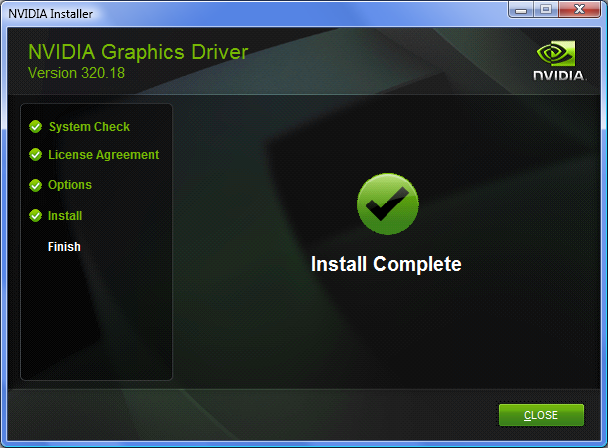### 性能对比

OpenCL_Sample.mq5 专为展示 MQL5 中使用 OpenCL 的诸多优势而编写。它会计算某些组中两个变量的函数值，并利用图形标签 (OBJ_BITMAP_LABEL) 在图表窗口中显示结果。有两种计算方式 - 使用 OpenCL 和不使用 OpenCL。而这些代码块也分别以 WithOpenCL() 和 WithoutOpenCL() 函数的形式实施：

```//+------------------------------------------------------------------+
//| Script program start function                                    |
//+------------------------------------------------------------------+
void OnStart()
{
//...
Print("\nCalculations without OpenCL:");
WithoutOpenCL(values1,colors1,w,h,size,const_1,const_2);
//--- calculations with OpenCL
Print("\nCalculations with OpenCL:");
WithOpenCL(values2,colors2,w,h,size,const_1,const_2);
//...
}
//+------------------------------------------------------------------+
//| Calculations without using  OpenCL                               |
//+------------------------------------------------------------------+
void WithoutOpenCL(float &values[],uint &colors[],const uint w,const uint h,
const uint size,const uint const_1,const uint const_2)
{
//--- store the calculation start time
uint x=GetTickCount();
//--- calculation of function values
for(uint i=0;i<h;i++)
for(uint j=0;j<w;j++)
values[i*w+j]=Func(InpXStart+i*InpXStep,InpYStart+j*InpYStep);
//--- print the function calculation time
Print("Calculation of function values = "+IntegerToString(GetTickCount()-x)+" ms");
//--- determine the minimum value and the difference between
//--- the minimum and maximum values of points in the set
float min,dif;
GetMinAndDifference(values,size,min,dif);
//--- store the calculation start time
x=GetTickCount();
//--- calculate paint colors for the set
uint a;
for(uint i=0;i<size;i++)
{
a=(uint)MathRound(255*(values[i]-min)/dif);
colors[i]=const_1*(a/16)+const_2*(a%16);
}
//--- print the paint color calculation time
Print("Calculation of paint colors = "+IntegerToString(GetTickCount()-x)+" ms");
}
//+------------------------------------------------------------------+
//| Calculations using OpenCL                                        |
//+------------------------------------------------------------------+
void WithOpenCL(float &values[],uint &colors[],const uint w,const uint h,
const uint size,const uint const_1,const uint const_2)
{
//--- variables for using OpenCL
int cl_ctx;
int cl_prg;
int cl_krn_1;
int cl_krn_2;
int cl_mem_1;
int cl_mem_2;
//--- create context for OpenCL (selection of device)
if((cl_ctx=CLContextCreate(CL_USE_ANY))==INVALID_HANDLE)
{
return;
}
//--- create a program based on the code in the cl_src line
if((cl_prg=CLProgramCreate(cl_ctx,cl_src))==INVALID_HANDLE)
{
CLContextFree(cl_ctx);
Print("OpenCL program create failed");
return;
}
//--- create a kernel for calculation of values of the function of two variables
if((cl_krn_1=CLKernelCreate(cl_prg,"Func"))==INVALID_HANDLE)
{
CLProgramFree(cl_prg);
CLContextFree(cl_ctx);
Print("OpenCL kernel_1 create failed");
return;
}
//--- kernel for painting points of the set in the plane
{
CLKernelFree(cl_krn_1);
CLProgramFree(cl_prg);
CLContextFree(cl_ctx);
Print("OpenCL kernel_2 create failed");
return;
}
//--- OpenCL buffer for function values
{
CLKernelFree(cl_krn_2);
CLKernelFree(cl_krn_1);
CLProgramFree(cl_prg);
CLContextFree(cl_ctx);
Print("OpenCL buffer create failed");
return;
}
//--- OpenCL buffer for point colors
{
CLBufferFree(cl_mem_1);
CLKernelFree(cl_krn_2);
CLKernelFree(cl_krn_1);
CLProgramFree(cl_prg);
CLContextFree(cl_ctx);
Print("OpenCL buffer create failed");
return;
}
//--- store the calculation start time
uint x=GetTickCount();
//--- array sets indices at which the calculation will start
uint offset={0,0};
//--- array sets limits up to which the calculation will be performed
uint work;
work=h;
work=w;
//--- calculation of function values
//--- pass the values to the kernel
CLSetKernelArg(cl_krn_1,0,InpXStart);
CLSetKernelArg(cl_krn_1,1,InpYStart);
CLSetKernelArg(cl_krn_1,2,InpXStep);
CLSetKernelArg(cl_krn_1,3,InpYStep);
CLSetKernelArgMem(cl_krn_1,4,cl_mem_1);
//--- start the execution of the kernel
CLExecute(cl_krn_1,2,offset,work);
//--- read the obtained values to the array
//--- print the function calculation time
Print("Calculation of function values = "+IntegerToString(GetTickCount()-x)+" ms");
//--- determine the minimum value and the difference between
//--- the minimum and maximum values of points in the set
float min,dif;
GetMinAndDifference(values,size,min,dif);
//--- store the calculation start time
x=GetTickCount();
//--- set the calculation limits
uint offset2={0};
uint work2;
work2=size;
//--- calculation of paint colors for the set
//--- pass the values to the kernel
CLSetKernelArg(cl_krn_2,0,min);
CLSetKernelArg(cl_krn_2,1,dif);
CLSetKernelArg(cl_krn_2,2,const_1);
CLSetKernelArg(cl_krn_2,3,const_2);
CLSetKernelArgMem(cl_krn_2,4,cl_mem_1);
CLSetKernelArgMem(cl_krn_2,5,cl_mem_2);
//--- start the execution of the kernel
CLExecute(cl_krn_2,1,offset2,work2);
//--- read the obtained values to the array
//--- print the paint color calculation time
Print("Calculation of paint colors = "+IntegerToString(GetTickCount()-x)+" ms");
//--- delete OpenCL objects
CLBufferFree(cl_mem_1);
CLBufferFree(cl_mem_2);
CLKernelFree(cl_krn_1);
CLKernelFree(cl_krn_2);
CLProgramFree(cl_prg);
CLContextFree(cl_ctx);
}
```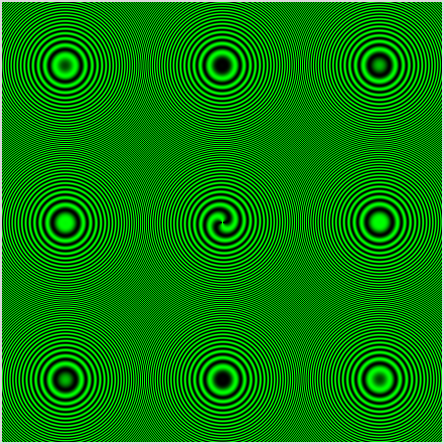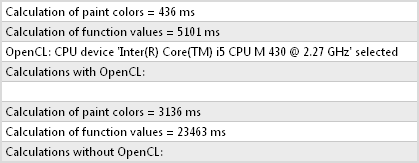OpenCL 设备

AMD Radeon HD 7970 20,361 毫秒
171 毫秒
119.07 倍
NVidia GeForce GT 630
24,742 毫秒
578 毫秒
42.8 倍
Intel Core i5 430M
27,222 毫秒
5,428 毫秒
5.01 倍
AMD Athlon X2 Dual-Core QL-65
45,723 毫秒
9,516 毫秒  4.8 倍

opencl_sample.mq5 (12.93 KB)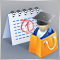“MQL5 应用商店” 2013 年一季度业绩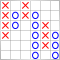点数图指标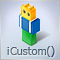基于自定义指标的交易信号生成器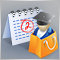MQL5 应用商店 2013 年二季度业绩